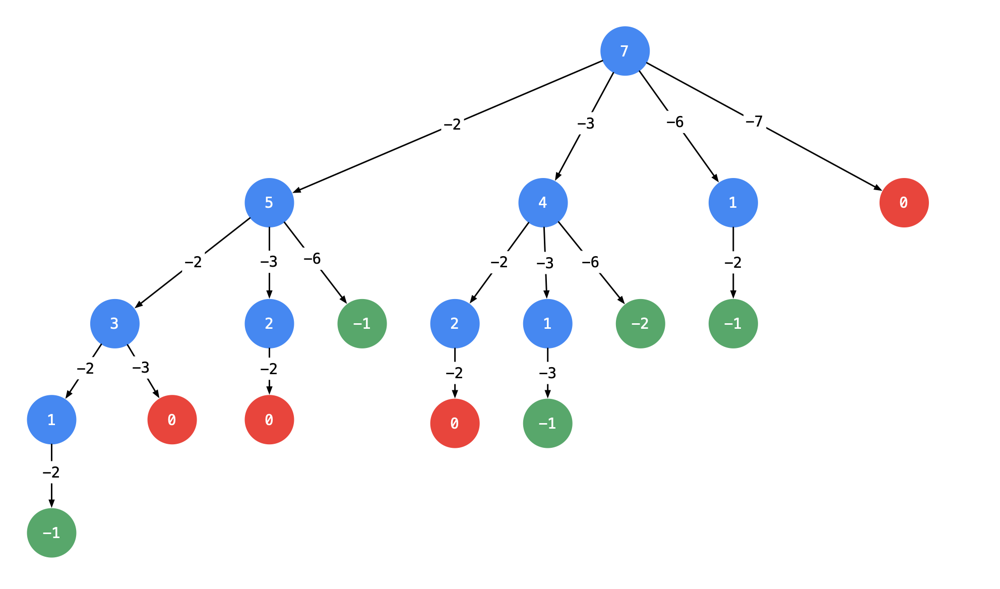By Long Luo

# Backtracking

It’s a typical Backtracking algorithm.

We can easily draw the tree below.Take $\textit{target} = 7$ as the root node and subtract the value of the edge while creating a child node.

Stop it when the value of the node is $0$ or a negative number.

All paths from the root node to leaf node $0$ are the results of the question.

Let’s coding.

Take $\textit{target} = 7$ for example, $[[2, 2, 3], [2, 3, 2], [3, 2, 2], ]$ are the answer.

However, the correct answer is $[, [2, 2, 3]]$.

Since it requires that each solution is not in order, so the $[2, 2, 3]$ and $[2, 3, 2]$ are the same answer.

What’s problem?

When and how did we duplicate the path?

# Why Duplicated?

At each node of the tree, all the candidates were searched and used, so there is a duplicate list.

Is it possible to deduplicate while searching?

Of course we can.

We have to search the elements in order while searching, since the output is not by order. We search from the certain position in the next round.

By now we deduplicating it and complete the problem.

# Pruning

We can prune the tree to make it work more efficiency.

## Analysis

• Time Complexity: $O(Sum)$, $Sum$ is all the path.
• Space Complexity: $O(\textit{target})$.

All suggestions are welcome.
If you have any query or suggestion please comment below.
Please upvote👍 if you like💗 it. Thank you:-)

Explore More Leetcode Solutions. 😉😃💗